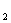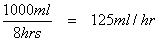## Table of Contents

1. Introduction
2. Calculating the number of tablets or volume of elixir
3. Calculating the volume for injections
4. Calculating IV Fluid Infusion Rates for Fluids with Gravity Feed Infusion Sets
5. Calculating drug infusion rates
6. Suggested reading

## Calculating IV Fluid Infusion Rates for Fluids with Gravity Feed Infusion Sets

When you need to calculate the number of drops per minute for an I.V. infusion rate, you need to know the following information:

1. Prescription giving volume of fluid to be infused and time over which it is to be given. e.g. 1 litre of 0.9% Sodium Chloride over 8 hours.
2. If you are using a gravity feed infusion set you will need to know the number of drops which make up 1 ml. This is written on the packaging.

N.B. normally 20 macrodrops = 1ml crystalloid e.g. 20 drops of HO = 1ml and 15 macrodrops = 1ml colloid

Then simply calculate the following;

1. The number of ml to be given per minute.
2. Multiply this figure by the number of drops in 1ml.

e.g. For 1 litre of 0.9% Sodium Chloride over 8 hours:2.08ml/min x 20 drops/ml = 41.6 drops per minute

This is closest to 42 drops per minute so you would run the infusion at a 42 drops per minute rate.